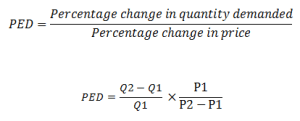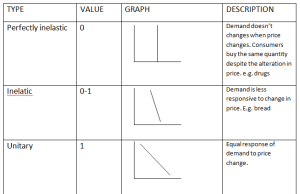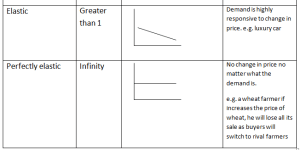Sunday , September 22 2019
ONLINE BOOK STORE (Shop Now)
Home / Igcse Economics Revision Notes / Price Elasticity Of Demand

# Price Elasticity Of Demand

Price Elasticity Of Demand, measures the responsiveness of demand to a change in price.

The formula used to calculate (PED) is:Q1 = Old Quantity

Q2 = New Quantity

P1 = Old Price

P2 = New Price

If the answer using the above formula is less than 1 than the product has price inelastic demand

however, if the answer is greater than 1 than the product has price elastic demand.

Price Elastic Demand: When demand changes by a greater percentage than the changes in price.

Price Inelastic Demand: When demand changes by a smaller percentage than the changes in price.

### Revenue Maximization By Using Price Elasticity Of Demand:

Revenue: Total reward of producing goods and services.

Formula:

• Price/unit × Quantity produced /demanded
• Total cost + Total profitThe above diagrams show that:

If demand is inelastic, producers must charge high prices in order to maximize revenue.

If demand is elastic, producers must charge low price in order to maximize revenue.

### Types Of Price Elasticity Of Demand:### Factors Affecting Price Elasticity Of Demand:

#### Availability Of Substitutes:

Substitutes more available      PED will be elastic

Less substitutes available         PED will be in elastic

#### Proportion Of Income Spent:

Small proportion (e.g. salt)        PED will be inelastic

Large proportion (e.g. car)         PED will be elastic

Nature Of Product:

Need (e.g. bread)       PED will be inelastic

Luxuries (e.g. car)       PED will be elastic

#### Addictive / Habit forming:

Cigarettes are addictive thus it will have inelastic PED.

#### Fashion and Trend:

In fashion             PED will be elastic

Out of fashion     PED will be inelastic## Characteristics And Functions Of Money

Money: Money is an item which is generally acceptable as a means of payment. Characteristics …

### One comment

1.Thank you so much for giving a good revision notes to me it is very helpful to me.